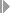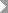Show TOC

###(06) POC Method on Basis of Revenue Planned by PeriodYou can use the POC Method on Basis of Revenue Planned by Period for the following:

• Projects

• Sales orders

• Internal orders with revenue

You can use the POC Method on Basis of Revenue Planned by Period to do the following:

• Calculate revenue affecting net income on the basis of the cumulative planned revenue

• Determine the costs affecting net income

• Determine the revenue in excess of billings

• Determine the capitalized costs

• Determine the reserves for unrealized costs

• Determine revenue surplus

• Report unrealized profits

The POC method is often used in Britain and North America. Since German law does not allow unrealized profits to be reported, the POC method is used in Germany only for internal information purposes.

#### Prerequisites

You have planned costs, revenues, and quantities.

Choose a results analysis method in simplified Customizing for Product Cost by Sales Order underPeriod-End ClosingResults AnalysisValuation Method.

#### Features

R(PA) = R(p) cumulative up to results analysis period

POC = R(PA) / R(p) total

C(PA) = POC * C(p) = R(PA) / R(p) * C(p)

The revenue affecting net income equals the cumulative planned revenue up to the result analysis period.

Costs affecting net income are calculated by dividing the revenue affecting net income by the total planned revenue multiplied by the total planned costs.

If actual costs are greater than or equal to calculated costs, the system creates capitalized costs.

If actual costs are less than calculated costs, the system creates reserves for unrealized costs.

If actual revenue is less than or equal to calculated revenue, the system creates revenue in excess of billings.

If actual revenue is greater than calculated revenue, the system creates a revenue surplus. The revenue surplus is basically a reserve.

Both calculated revenue and calculated costs can be settled to CO-PA.

Capitalized costs, reserves for unrealized costs, revenue in excess of billings, and revenue surplus can be transferred to FI and EC-PCA when you settle.

#### Example

Suppose you have planned costs of USD 120,000 for your project.

You have planned revenues for each period as follows:

Period 01

Period 02

Period 03

Period 04

Revenues

50,000

40,000

60,000

50,000

Total planned revenue is USD 200,000.

##### Period 01

In period 01 you have actual costs of USD 20,000. You have not billed the customer. In results analysis, the system calculates the following data:

• Revenue affecting net income of USD 50,000

• Costs affecting net income of USD 30,000

• Reserves for unrealized costs of USD 10,000

• Revenue in excess of billings of USD 50,000

You then settle the following:

• Calculated costs and revenues to CO-PA

• Reserves for unrealized costs and revenue in excess of billings to FI and EC-PCA

The following values are reported in CO-PA:

 Calculated revenue 50,000 Calculated cost of sales 30,000 Profit 20,000

Capitalized profit is shown in the balance sheet. Definitions:

Revenue in Excess of Billings = Capitalized Costs + Capitalized Profit

In this example, capitalized costs are USD 20,000.

Capitalized profit is USD 30,000.

Because reserves of USD 10,000 are shown as a liability, the total unrealized profit capitalized is USD 20,000.

The income statement shows the following values:

Income Statement

Expense

Revenue

Actual costs 20,000

Revenue in excess of billings 50,000

Reserves for unrealized costs 10,000

Profit 20,000

50,000

50,000

##### Period 02

In period 02 actual costs increase to USD 80,000. You deliver to your customer and send him a milestone invoice for USD 100,000. The order is partially delivered and partially billed. In results analysis, the system calculates the following data:

• Revenue affecting net income of USD 90,000

• Costs affecting net income of USD 54,000

• Capitalized costs of USD 26,000

• Surplus revenue of USD 10,000

You then settle the following:

• Calculated revenue and calculated costs to CO-PA

• Capitalized costs and revenue surplus to FI and EC-PCA

The following values are reported in CO-PA:

 Calculated revenue 90,000 Calculated cost of sales 54,000 Profit 36,000

The income statement shows the following values:

Income Statement

Expense

Revenue

Actual costs 80,000

Actual revenue 100,000

Revenue surplus 10,000

Capitalized costs 26,000

Profit 36,000

126,000

126,000

##### Period 03

In period 03 actual costs increase to USD 90,000. You deliver a second amount to your customer and send him a second milestone billing for USD 90,000. Total revenue is USD 190,000. The invoiced quantity is 40 units. The order is partially delivered and partially billed. In results analysis, the system calculates the following data:

• Revenue affecting net income of USD 150,000

• Costs affecting net income in the amount of the actual costs of USD 90,000

• Surplus revenue of USD 40,000

You then settle the following:

• Calculated revenue and calculated costs to CO-PA

• Revenue surplus to FI and EC-PCA

The following values are reported in CO-PA:

 Calculated revenue 150,000 Calculated cost of sales 90,000 Profit 60,000

The income statement shows the following values:

Income Statement

Expense

Revenue

Actual costs 90,000

Actual revenue 190,000

Revenue surplus 40,000

Profit 60,000

190,000

190,000

##### Period 04

In period 04 actual costs increase to USD 130,000. You deliver the remaining goods and send the customer the final invoice for USD 10,000. Total revenue is USD 200,000. The invoiced quantity is 20 units. The order is fully delivered and fully billed.

In results analysis, the system calculates the following data:

• Costs affecting net income in the amount of the actual costs of USD 130,000

• Revenue affecting net income of USD 200,000 Calculated revenue is set equal to actual revenue.

You then settle the following:

• Calculated revenue and actual costs to CO-PA

• The cancellation of the revenue surplus to FI and EC-PCA

The following values are reported in CO-PA:

 Calculated revenue 200,000 Calculated cost of sales 130,000 Profit 70,000

The income statement shows the following values:

Income Statement

Expense

Revenue

Actual costs 130,000

Actual revenue 200,000

Profit 70,000

200,000

200,000

The project has a total profit of USD 70,000.